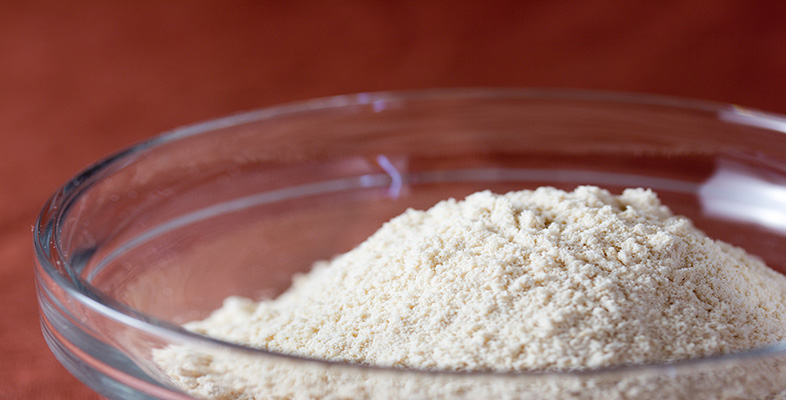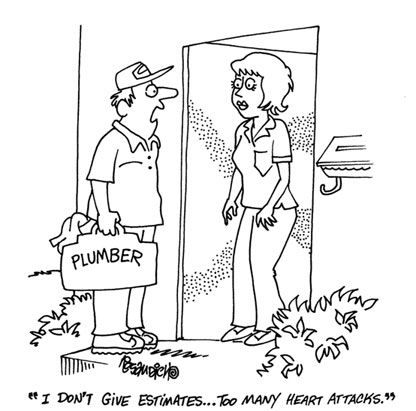### Become an OU studentRounding and estimation

Start this free course now. Just create an account and sign in. Enrol and complete the course for a free statement of participation or digital badge if available.

# 3.4 Did I make a rough estimate to act as a check?When using a calculator many people have ‘blind faith’ in its capacity to provide the correct result.

Calculators invariably provide the correct result for the information they are given; any errors are due to the operator.

To help guard against errors, always give a ‘ball-park’ estimate for a problem, using rounded values and easy calculations.

For example, make a rough estimate of the calculation below to act as a check upon the actual calculation using the calculator.

3.421 + 5.986 + 12.009 87 = ?

Rough check: 3 + 6 + 12 = 21

The two answers are within the same ‘ball-park’ so you should be happy with the calculator's answer. Had you got an answer of 3438.995 87, say, you should be suspicious and checking would reveal you had missed out a decimal point.

It is helpful to adopt this checking strategy for all your calculations since it is so easy to press the wrong key inadvertently, or double press a key when working at speed on a calculator.

Often it is useful to have both an underestimate and an overestimate.

## Example 11

Look back at Example 9, which gave an incorrect answer. How could you have spotted this without doing the whole calculation?

First do an overestimate, then an underestimate, per person.

In an overestimate, ignore the float and round the food up to £40, drinks to £6, taxi £4, tip £3 minus £5 discount gives £48 this is less than £50. Divide by 5 people means the maximum share for each person is £10. Hence £10.80 is too much.

An underestimate would be £35 for food, £5 for drinks, £3 for taxi and £3 for tip, less £5 for the voucher and £1 float. This gives £40, divided by 5, which means minimum of £8 each.

Hence you would expect an answer between £8 and £10.

One common error when using a calculator is to forget that the calculator does not total everything as it goes along. So, for example, in the case of the bill for a night out, you must total everything before you divide by the number of people who are sharing the bill.

## Example 12

Look back at Example 8 and suppose that the calculation was put into a scientific calculator, in the following form:

0.04 + 5.00 + 8.00 − 36.98 − 5.10 − 3.50 − 3.00 ÷ 4 = ?

Would this give the right answer?